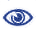# Prague Stringology Conference 2014

On the Number of Distinct Squares

 Abstract: Counting the number of types of squares rather than their occurrences, we consider the problem of bounding the maximum number of distinct squares in a string. Fraenkel and Simpson showed in 1998 that a string of length n contains at most 2n distinct squares and indicated that all evidence pointed to n being a natural universal upper bound. Ilie simplified the proof of Fraenkel-Simpson's key lemma in 2005 and presented in 2007 an asymptotic upper bound of 2nΘ(log n). We show that a string of length n contains at most ⌊11n/6⌋ distinct squares for any n. This new universal upper bound is obtained by investigating the combinatorial structure of FS-double squares (named so in honour of Fraenkel and Simpson's pioneering work on the problem), i.e. two rightmost-occurring squares that start at the same position, and showing that a string of length n contains at most ⌊5n/6⌋ FS-double squares. We will also discuss a much more general approach to double-squares, i.e. two squares starting at the same position and satisfying certain size conditions. A complete, so-called canonical factorization of double-squares that was motivated by the work on the number of distinct squares is presented in a separate contributed talk at this conference. The work on the problem of the number of distinct squares is a joint effort with Antoine Deza and Adrien Thierry.

 Download paper:PostScript PDF BibTeX reference
 Download presentation: